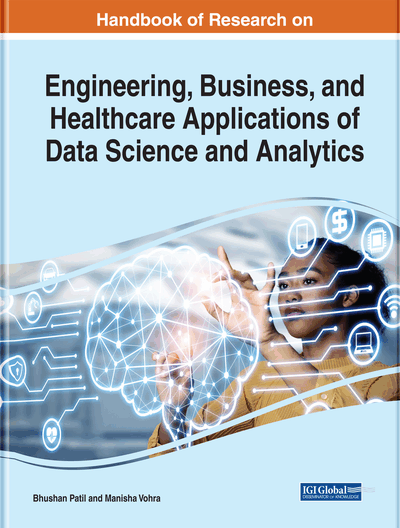# Machine Learning and Its Application in Monitoring Diabetes Mellitus

Vandana Kalra, Indu Kashyap, Harmeet Kaur
DOI: 10.4018/978-1-7998-3053-5.ch012
OnDemand:
(Individual Chapters)
Available
\$29.50
No Current Special Offers

## Abstract

Data science is a fast-growing area that deals with data from its origin to the knowledge exploration. It comprises of two main subdomains, data analytics for preparing data, and machine learning to probe into this data for hidden patterns. Machine learning (ML) endows powerful algorithms for the automatic pattern recognition and producing prediction models for the structured and unstructured data. The available historical data has patterns having high predictive value used for the future success of an industry. These algorithms also help to obtain accurate prediction, classification, and simulation models by eliminating insignificant and faulty patterns. Machine learning provides major advancement in the healthcare industry by assisting doctors to diagnose chronic diseases correctly. Diabetes is one of the most common chronic disease that occurs when the pancreas cells are damaged and do not secrete sufficient amount of insulin required by the human body. Machine learning algorithms can help in early diagnosis of this chronic disease by studying its predictor parameter values.
Chapter Preview
Top

## Issues, Controversies, Problems

Few challenges (Sendak, M.et al.2019) in relation to healthcare are listed below which can be handled using appropriate machine learning algorithms. In the case study provided, one of the challenge of identifying chronic disease of diabetes at early stages is explained in detail using machine learning algorithm K- nearest neighbour.

## Key Terms in this Chapter

Confusion Matrix: A confusion matrix is a two-dimensional table depicting summary of classification or prediction outcomes produced by applying classification algorithm on the given dataset. The accurate and inaccurate predictions produced with respect to each class are summarized with their count values. It gives the insight of the errors being made during classification process by a classifier.

Clustering: Clustering is a type of unsupervised learning technique where data objects are placed into different collections called clusters, based on their degree of dissimilarity. All like data objects are a part of the same cluster.

Machine Learning: Machine learning is a scientific approach to analyse available data using algorithms and statistical models to accomplish a specific task by utilizing the patterns evolved.

Diabetes Miletus: It is a chronic disease in which the body’s capability to produce or react to the insulin hormone is reduced. This effects absorption of carbohydrates and also raised the glucose level in the blood.

Data Science: It is a science of multiple disciplines used for exploring knowledge from data using complex scientific algorithms and methods.

Artificial Neural Network: Artificial neural network is a collection of connected input/output units called neurons. Each connection has a weight associated with it to develop and test computational analysis of neurons. This neural network learns by adjusting these weights iteratively till it is able to predict the correct class label of the input data.

Classification: Classification is the scientific procedure for predicting the class label or category of given test data objects. Classification is also a task of predictive modelling in which a mapping function is approximated using inputted feature variables to produce output target variable.

F-Score: F-score is a statistical measure which expresses the balance between the precision and the recall. It considers both the precision p and the recall r of the test to compute accuracy. It is the harmonic mean of the precision and recall with its best value at 1 and worst at 0.

Precision: It is an accuracy measure calculated as the ratio of number of true positives predictions to the total of number of true positives and the number of false positives predictions. True positive cases are those cases which the classifier correctly labelled a data point as positive whereas false positive predictions are cases in which a data point is incorrectly labelled as positive by the classifier that are actually negative.

Accuracy: It is the ratio of number of accurate predictions produced by classification algorithm to the total number of input data samples.

Recall: It is an accuracy measure calculated as ratio of the number of true positives predictions to the total of number of true positives and the number of false negatives. True positives cases are those cases where data point is classified as positive by the classifier that actually are positive and false negatives are cases with such data points that are identified as negative by classifier but actually are positive.

## Complete Chapter List

Search this Book:
Reset